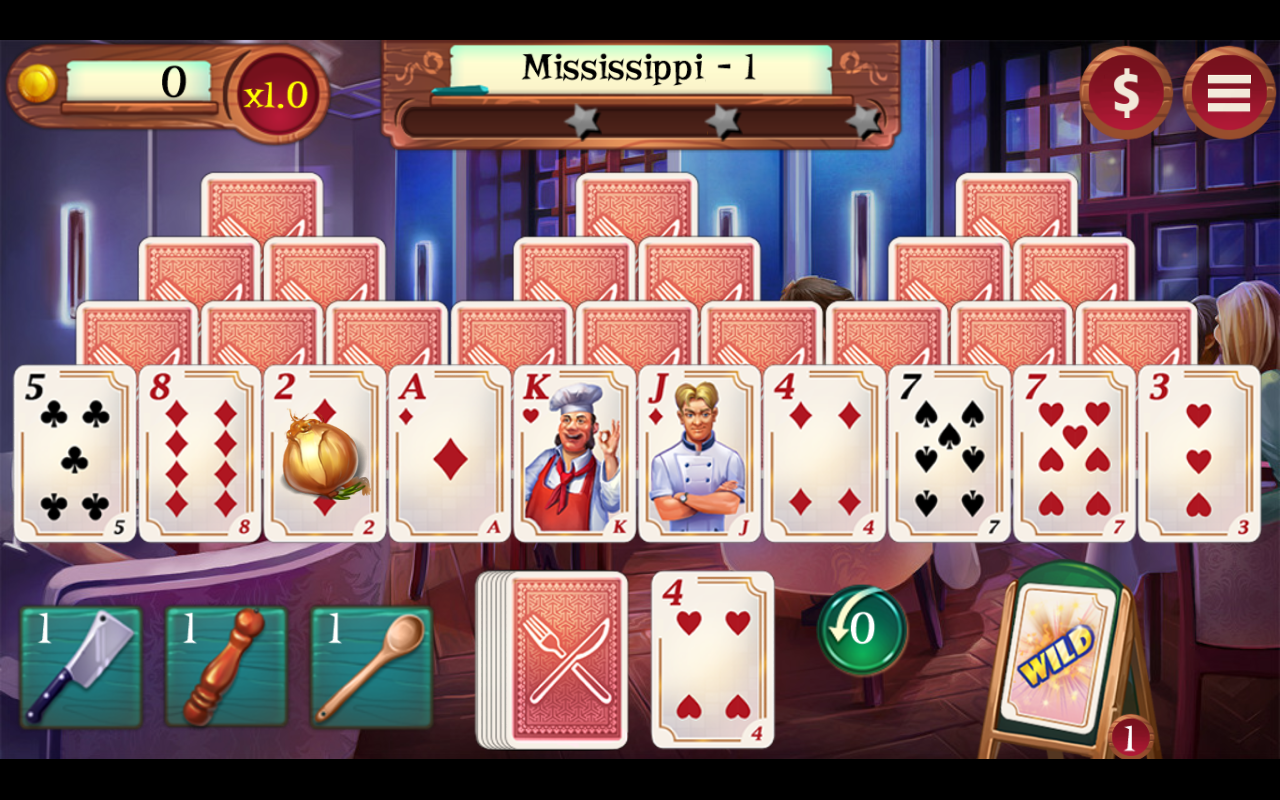-=+=- -=+=- -=+=- -=+=- -=+=- -=+=- -=+=- -=+=- -=+=- -=+=- -=+=- -=+=- -=+=- -=+=- -=+=- -=+=- -=+=- -=+=- -=+=- -=+=- -=+=- -=+=- -=+=- -=+=- -=+=- -=+=- -=+=- -=+=- -=+=- -=+=- (c) WidthPadding Industries 1987 0|433|0 -=+=- -=+=- -=+=- -=+=- -=+=- -=+=- -=+=- -=+=- -=+=- -=+=- -=+=- -=+=- -=+=- -=+=- -=+=- -=+=- -=+=- -=+=- -=+=- -=+=- -=+=- -=+=- -=+=- -=+=- -=+=- -=+=- -=+=- -=+=- -=+=- -=+=-
SoCoder -> Showcase Home -> Puzzle

therevillsgamesCreated : 18 April 2016
Edited : 18 April 2016
System : Android
Language : Monkey

### Chef Solitaire: USA TriPeaks

Play TriPeaks Chef Style!

ScreenshotsPlay Chef Solitaire USA TriPeaks and put your chef hat on!
Open your very own restaurant and make it a scrumptious success. Enjoy TriPeaks solitaire to your heart's content and bring your hamburger baking skills to perfection. The stars you earn will open up new opportunities all around the USA. Cook up a solitaire feast with Chef Solitaire - USA today!

* Build a solitaire fuelled restaurant empire in the USA